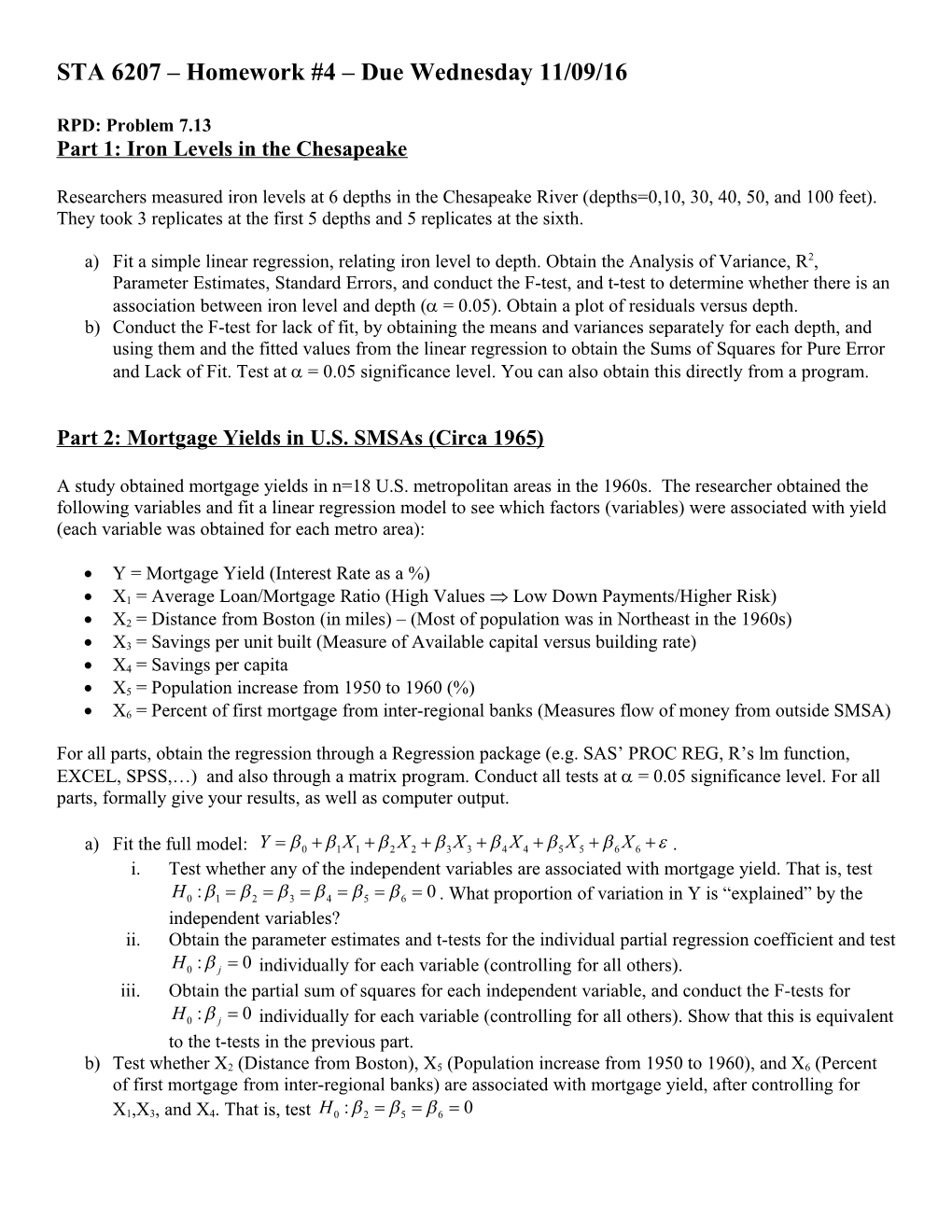# STA 6207 Homework #4 Due Wednesday 11/09/16STA 6207 – Homework #4 – Due Wednesday 11/09/16

RPD: Problem 7.13

Part 1: Iron Levels in the Chesapeake

Researchers measured iron levels at 6 depths in the Chesapeake River (depths=0,10, 30, 40, 50, and 100 feet). They took 3 replicates at the first 5 depths and 5 replicates at the sixth.

a)Fit a simple linear regression, relating iron level to depth. Obtain the Analysis of Variance, R2, Parameter Estimates, Standard Errors, and conduct the F-test, and t-test to determine whether there is an association between iron level and depth ( = 0.05). Obtain a plot of residuals versus depth.

b)Conduct the F-test for lack of fit, by obtaining the means and variances separately for each depth, and using them and the fitted values from the linear regression to obtain the Sums of Squares for Pure Error and Lack of Fit. Test at  = 0.05 significance level. You can also obtain this directly from a program.

Part 2: Mortgage Yields in U.S. SMSAs (Circa 1965)

A study obtained mortgage yields in n=18 U.S. metropolitan areas in the 1960s. The researcher obtained the following variables and fit a linear regression model to see which factors (variables) were associated with yield (each variable was obtained for each metro area):

• Y = Mortgage Yield (Interest Rate as a %)
• X1 = Average Loan/Mortgage Ratio (High Values  Low Down Payments/Higher Risk)
• X2 = Distance from Boston (in miles) – (Most of population was in Northeast in the 1960s)
• X3 = Savings per unit built (Measure of Available capital versus building rate)
• X4 = Savings per capita
• X5 = Population increase from 1950 to 1960 (%)
• X6 = Percent of first mortgage from inter-regional banks (Measures flow of money from outside SMSA)

For all parts, obtain the regression through a Regression package (e.g. SAS’ PROC REG, R’s lm function, EXCEL, SPSS,…) and also through a matrix program. Conduct all tests at  = 0.05 significance level. For all parts, formally give your results, as well as computer output.

a)Fit the full model: .

1. Test whether any of the independent variables are associated with mortgage yield. That is, test . What proportion of variation in Y is “explained” by the independent variables?
2. Obtain the parameter estimates and t-tests for the individual partial regression coefficient and test individually for each variable (controlling for all others).
3. Obtain the partial sum of squares for each independent variable, and conduct the F-tests for individually for each variable (controlling for all others). Show that this is equivalent to the t-tests in the previous part.

b)Test whether X2 (Distance from Boston), X5 (Population increase from 1950 to 1960), and X6 (Percent of first mortgage from inter-regional banks) are associated with mortgage yield, after controlling for X1,X3, and X4. That is, test

Part 3: LPGA 2008 – Regression Analysis – Model Development

The dataset lpga1.dat contains statistics for the 2008 Ladies Professional Golf Association, containing the following variables:

• Golfer
• X1 = Number of Rounds
• X2 = Average Distance for Drives (Yards)
• X3 = Percent of Fairways hit
• X4 = Percent of Time on green in regulation
• X5 = Average number of putts per round
• X6 = Average number of sand traps hit per round
• X7 = Percent of time making par when in sand
• Y = ln(Prize Winnings per round (\$))

2)Obtain the best models with p’=2,…,8 in terms of R2, Adj-R2, CP, AIC,SBC (BIC in R).

3)Plot each of these versus p’.

4)Which model do you select?

5)Run the stepwise regression:

• If using SAS: with significance levels to stay and enter (sls=.15, sle=.15). What model is selected? Print out the results of this analysis.
• If using R, based on using minimum BIC criterion

6)RPD: 7.1, 7.2, 7.3, 7.4, 7.13

Use your best model from the lpga1.dat dataset (part 4) on lpga2.dat to validate the model. Use the model set up in Example 7.9 to:

1. Obtain Predicted values for lpga2 dataset, based on the regression from the lpga1 dataset (be sure and use (natural) logarithm of Prize Winnings.
2. Obtain  = P-Y for each of the golfers, as well as the mean and sd of 
3. Conduct the t-test of H0: Bias is 0 at  = 0.05 significance level.
4. Obtain the Mean Squared Error of Prediction (MSEP)
5. What proportion of MSEP is due to bias in the predicted values?